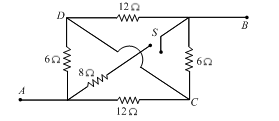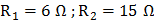# The equivalent resistance between pointsandwith switch S open and closed are respectivelya)b)c)d)## Question ID - 151321 :- The equivalent resistance between pointsandwith switch S open and closed are respectivelya)b)c)d)3537

(b)When switch S is open, the corresponding equivalent circuit diagram is as shown in the figureThe equivalent resistance betweenandisWhen switch S is closed, the corresponding equivalent circuit diagram is as shown in the figure belowThe equivalent resistanceandisNext Question :

In the Wheatstone bridge shown below, in order to balance the bridge, we must havea)b)c)any finite value d)any finite value